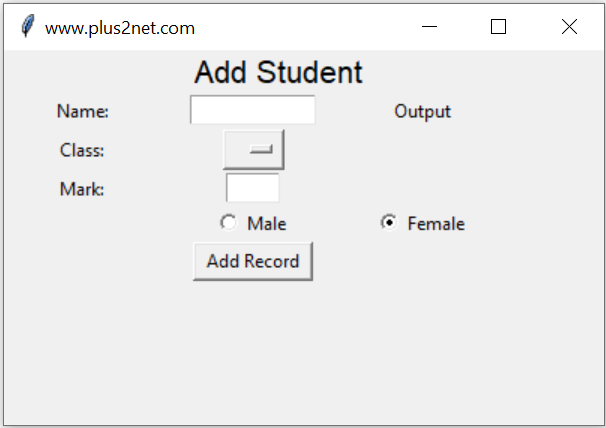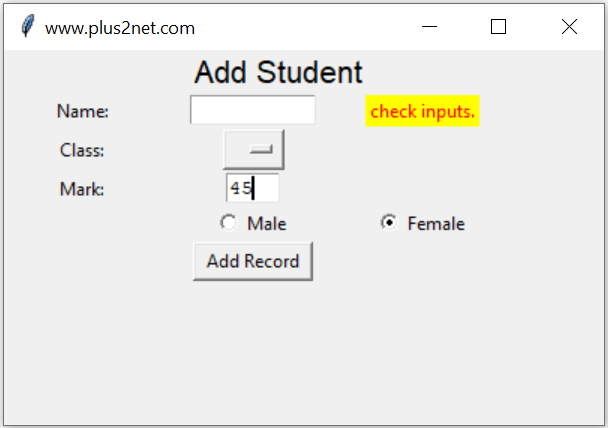# Inserting Data to MySQL at Webpage

Inserting data from TKinter GUI to web page using request

We are submitting user inputs from Tkinter window to a webpage by using request. We will submit data using a post method. Here is a sample data submission and getting message from website.
``````import requests
# url = "https://www.plus2net.com/python/tkinter-post-return.php"
url = "http://localhost/plus2net/python/tkinter-post-return.php"
data = {"name": "Alex", "class": "Four", "mark": 55, "gender": "Female"}
my_return = requests.post(url, data=data)
print(my_return.text)``````
To install request library.
``pip install requests``

## Target URL as demo

If you want to use the same target URL as shown above, on success you will get return message with the input name and one fixed id. Say if your name input is Alex then you will get return text as this.
``Alex id=55``
Here is the sample PHP script which receives the POST data and insert the same into student table.

``````<?Php
\$host_name = "localhost";
\$database = "database_name"; // Change your database name

try {
} catch (PDOException \$e) {
print "Error!: " . \$e->getMessage() . "";
die();
}
// database connection is over ///

\$name=\$_POST['name']; // Collect name
\$class=\$_POST['class'];
\$mark=\$_POST['mark'];
\$gender=\$_POST['gender'];

\$status='T';// fixing status vlaue for validation
\$query="insert into student(name,class,mark,gender)
values(:name,:class,:mark,:gender)";

\$step=\$dbo->prepare(\$query);
\$step->bindParam(':name',\$name,PDO::PARAM_STR, 15);
\$step->bindParam(':class',\$class,PDO::PARAM_STR, 15);
\$step->bindParam(':mark',\$mark,PDO::PARAM_INT,3);
\$step->bindParam(':gender',\$gender,PDO::PARAM_STR,10);

if(\$step->execute()){
\$mem_id=\$dbo->lastInsertId();
echo " \$name id = \$mem_id ";
}
else{
}
?>``````There are three parts in this script.
1. Part 1: Create the GUI window using tkinter.
2. Part 2: Validate the user entered data.
3. Part 3: Post data to webpage using request.

## Part 1 : Tkinter window to accept user inputs

User will enter four input data using our Tkinter window. Main components are here

Name : String of name through a text box t1
Class : Select one option from the dropdown OptionMenu
Mark : Integer value ( number ) through text box t3
gender : Selection through a pair of radio buttons ( Male , Female or other )
Submit : Button to trigger a click event to handle all the inputs.

## Using Request

``import requests``

There are Labels to show message about the input data ( l1,l2,l3 ) and one final Label ( l5 ) to display the output or return id of the record after inserting the data in table. One OptionMenu ( opt1) is used for selection of class.
``````l0 = tk.Label(my_w, text="Add Student", font=16, width=30, anchor="c")
l0.grid(row=1, column=1, columnspan=4)

l1 = tk.Label(my_w, text="Name: ", width=10, anchor="c")
l1.grid(row=3, column=1)

t1 = tk.Text(my_w, height=1, width=10, bg="white")
t1.grid(row=3, column=2)

l2 = tk.Label(my_w, text="Class: ", width=10)
l2.grid(row=4, column=1)

# add list box for selection of class
options = StringVar(my_w)
options.set("")  # default value

opt1 = OptionMenu(my_w, options, "Three", "Four", "Five")

l3 = tk.Label(my_w, text="Mark: ", width=10)
l3.grid(row=5, column=1)

t3 = tk.Text(my_w, height=1, width=4, bg="white")
t3.grid(row=5, column=2)

r2.grid(row=6, column=2)

r3.grid(row=6, column=3)

b1 = tk.Button(my_w, text="Add Record", width=10,
b1.grid(row=7, column=2)
my_str = tk.StringVar()
l5 = tk.Label(my_w, textvariable=my_str, width=10)
l5.grid(row=3, column=3)
my_str.set("Output")``````
The Button b1 is used to execute the function add_data() by using the command or click event of the button.
``````b1 = tk.Button(my_w,  text='Add Record', width=10,
b1.grid(row=7,column=2)``````
Inside the function add_data() we have two sections. In first section we will check each input data. It is expected that no input is left blank by user. Without filling the details if user has clicked the button ( b1 ) then we will display a message through our Label ( l5 ) asking to check the inputs ( Read the else part of the if-else).
``````def add_data():
flag_validation=True # set the flag

# length of my_name , my_class and my_gender more than 2
if(len(my_name) < 2 or len(my_class)<2  or len(my_gender) < 2 ):
flag_validation=False
try:
val = int(my_mark) # checking mark as integer
except:
flag_validation=False

if(flag_validation):
#  Part 3  Send the request with data to target URL
else:
l5.config(fg='red')   # foreground color
l5.config(bg='yellow') # background color
my_str.set("check inputs.")``````In this code we first set one flag validation ( flag_validation ) to True, if any where the validation of the inputs fails then we will change this status of validation to False. ( this is part of the above code )
``````if(len(my_name) < 2 or len(my_class)<2  or len(my_gender) < 2 ):
flag_validation=False
try:
val = int(my_mark) # checking mark as integer
except:
flag_validation=False ``````
After all the checks ( validation of inputs ) if the flag ( flag_validation ) is True , then we can insert data to Excel file and save the file.
``````    if flag_validation:
# url = "https://www.plus2net.com/python/tkinter-post-return.php"
url = "http://localhost/plus2net/python/tkinter-post-return.php"
data = {
"name": my_name,
"class": my_class,
"mark": my_mark,
"gender": my_gender,
}
my_return = requests.post(url, data=data)
print(my_return.text)
t1.delete("1.0", END)  # reset the text entry box
t3.delete("1.0", END)  # reset the text entry box
l5.config(fg="green")
l5.config(bg="white")
my_str.set(my_return.text)

l5.after(2000, lambda: my_str.set(""))``````

We want to show the ID ( after adding the record ) or the error message for some time ( say 3 seconds ) and it should vanish after the time delay.
``l5.after(3000,lambda:l5.config(fg='white',bg='white',text=''))``
``````import tkinter as tk
from tkinter import *

import requests

my_w = tk.Tk()
my_w.geometry("400x250")
my_w.title("www.plus2net.com")

l0 = tk.Label(my_w, text="Add Student", font=16, width=30, anchor="c")
l0.grid(row=1, column=1, columnspan=4)

l1 = tk.Label(my_w, text="Name: ", width=10, anchor="c")
l1.grid(row=3, column=1)

t1 = tk.Text(my_w, height=1, width=10, bg="white")
t1.grid(row=3, column=2)

l2 = tk.Label(my_w, text="Class: ", width=10)
l2.grid(row=4, column=1)

# add list box for selection of class
options = StringVar(my_w)
options.set("")  # default value

opt1 = OptionMenu(my_w, options, "Three", "Four", "Five")

l3 = tk.Label(my_w, text="Mark: ", width=10)
l3.grid(row=5, column=1)

t3 = tk.Text(my_w, height=1, width=4, bg="white")
t3.grid(row=5, column=2)

r2.grid(row=6, column=2)

r3.grid(row=6, column=3)

b1.grid(row=7, column=2)
my_str = tk.StringVar()
l5 = tk.Label(my_w, textvariable=my_str, width=10)
l5.grid(row=3, column=3)
my_str.set("Output")

flag_validation = True
my_name = t1.get("1.0", END)  # read the name
my_class = options.get()  # read class
my_mark = t3.get("1.0", END)  # read mark

if len(my_name) < 2 or len(my_class) < 2 or len(my_gender) < 2:
flag_validation = False
try:
val = int(my_mark)  # checking mark as integer
except:
flag_validation = False
if flag_validation:
url = "https://www.plus2net.com/python/tkinter-post-return.php"
#url = "http://localhost/plus2net/python/tkinter-post-return.php"
data = {
"name": my_name,
"class": my_class,
"mark": my_mark,
"gender": my_gender,
}
my_return = requests.post(url, data=data)
print(my_return.text)
t1.delete("1.0", END)  # reset the text entry box
t3.delete("1.0", END)  # reset the text entry box
l5.config(fg="green")
l5.config(bg="white")
my_str.set(my_return.text)

l5.after(2000, lambda: my_str.set(""))
else:
l5.config(fg="red")
l5.config(bg="yellow")
my_str.set("Check Inputs")
l5.after(2000, lambda: my_str.set(""))

my_w.mainloop()
``````

Subscribe to our YouTube Channel here

## Subscribe

* indicates required
Subscribe to plus2netplus2net.com